This article explains the galaxy generation algorithm showcased in the video below. It is written in C#, rendered with OpenGL and the source code is available here.

At the end of the article you can see how the galaxy is used as the server selection screen in our game Sector’s Edge, as well as some extreme examples.

Galaxy Anatomy

Each galaxy is composed of an axis and multiple arms: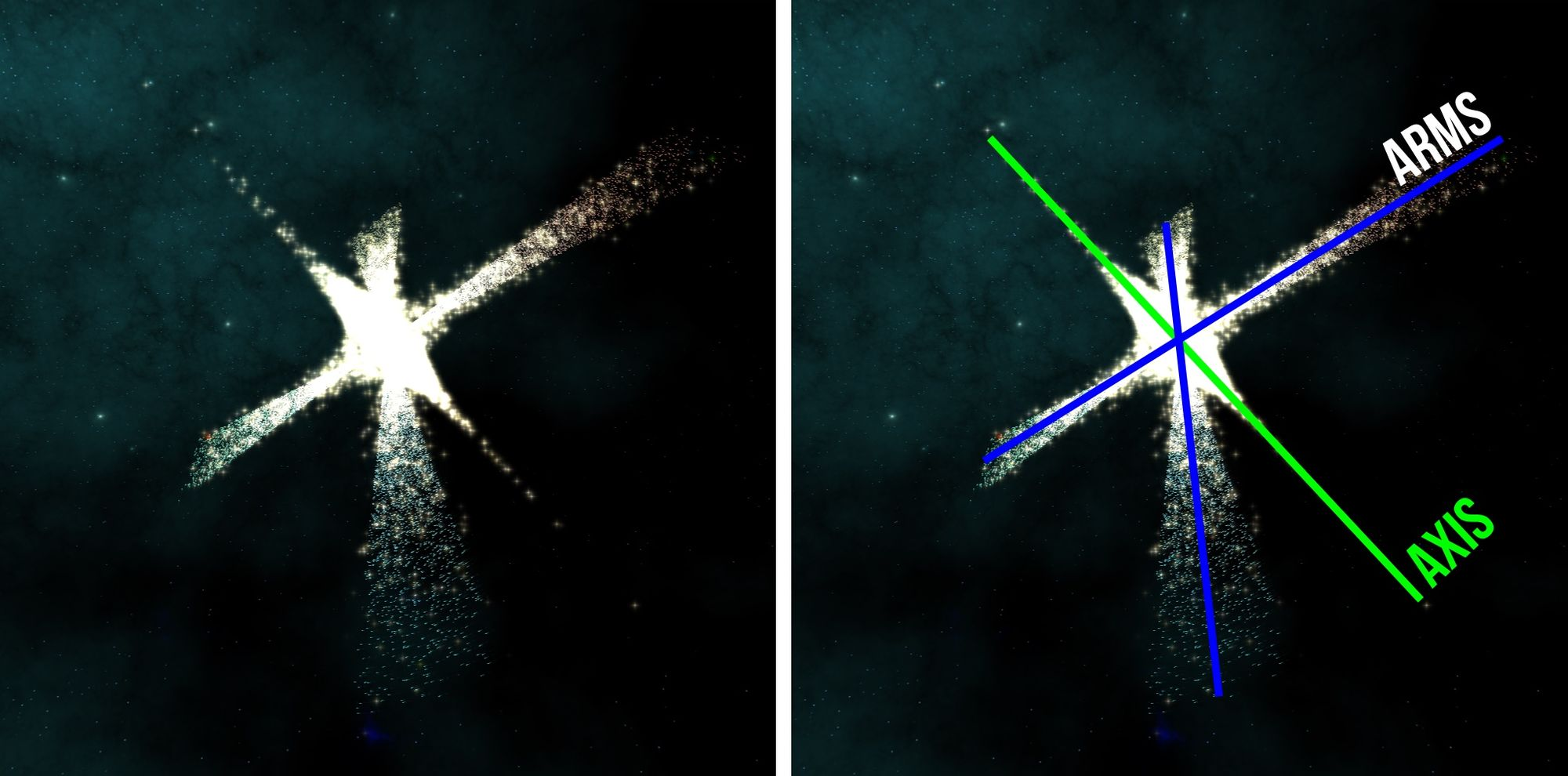The arms of this galaxy are straight for visual clarity

The generation process follows the following stages:

• Arm generation
• Axis generation
• Arm scaling
• Arm bending
• Height variance
• Applying colour
• Creating multiple arm layers

Part 1 - Generating the Arms

The code below produces multiple flat arms and is controlled by the following parameters:

• gravity — pushes points towards the center of the galaxy so that the galaxy will appear brighter and more densely populated towards the center
• galaxySize — controls the radius of the galaxy
• armCount— controls the amount of arms
• armSpread — controls the width of each arm
Vector3 GetPoint()
{
Vector3 v;
double armDivisor = Math.PI / armCount;

while (true)
{
// Generate a random normalised point with a weighting
// towards the center controlled by the gravity variable
v = NextV3(-1, 1).Normalized() * Math.Pow(Next(0, 1.0), gravity);

// Some points will not conform to the parameters
if (random.NextDouble() > conformChance)
break;

bool valid = false;

// Calculate the global angle of the point around the axis
var d = Math.Atan2(v.X, v.Z);

// Check if this point lands on an arm
for (double j = -Math.PI; j <= Math.PI; j += armDivisor)
{
// A point lands on an arm if its distance
// from the start of the arm is less than angle * armDistance
if (d > j && d < j + armDivisor * armSpread)
{
valid = true;
break;
}
}

if (valid)
break;
}

v.Y = 0;
return v * galaxySize;
}

Part 2 - Generating the Axis

The axis is composed of stars, which are generated in the same manner as the points in the arms. Stars closer to the center of the galaxy are shifted vertically proportional to the beamHeight parameter.

var v = GetPoint();

// Add some variance to each star
v += NextV3(-0.5, 0.5);

// Shift stars vertically as they get closer to the center of the galaxy
var s = beamHeight / v.Magnitude;
v.Y = Next(-s, s);

Stars are rendered as points into a framebuffer and then modified with a Gaussian blur to mimic a diffraction spike.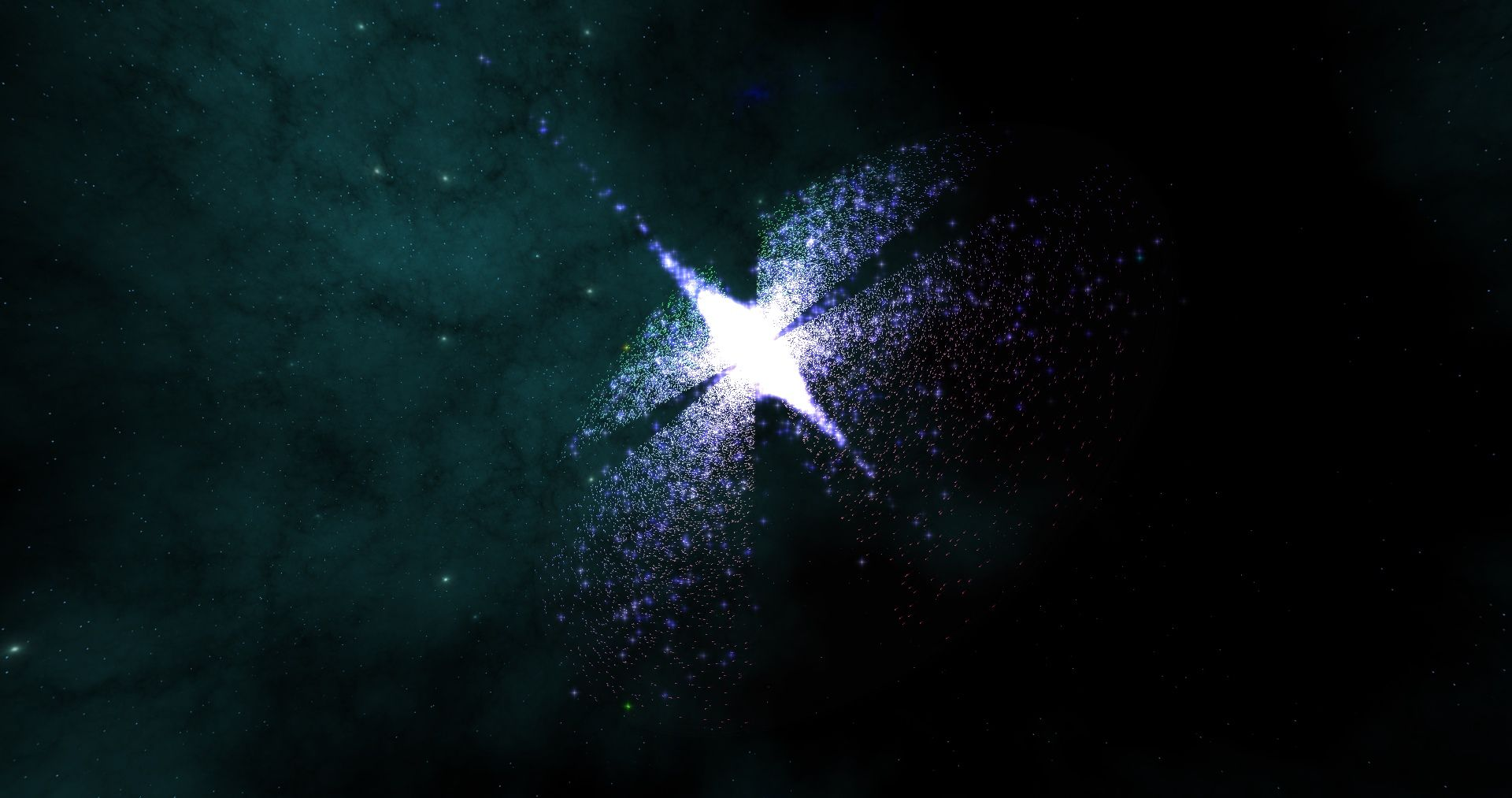A flat galaxy populated with stars

Part 3 - Arm Scaling

In a generated galaxy, some arms can be longer than others. The diagram below represents a top-down view of the galaxy. Any points that land inside the scaling region are extended further outwards.

θ¹ represents the scalingAngleStart parameter and θ² represents the scalingAngleEnd parameter. Any points that lie within the scaling region are extended outwards proportional to the scalingPower parameter. This calculation is added to the end of the GetPoint() function:

Vector3 GetPoint()
{
Vector3 v = Vector3.Zero;

// Generate a point
...

// Calculate the global angle of the point around the axis
var a = Math.Atan2(v.X, v.Z);

// Calculate the angle of this point inside its quadrant
var e = Math.Abs(Math.Abs(a) - Math.PI / 2);

var magnitude = galaxySize;

// Scale the point outwards if it lands within the scaling region
if (e > scalingAngleStart && e < scalingAngleEnd)
magnitude = magnitude * 2 / Math.Pow(e, scalingPower);

v.X *= magnitude;
v.Z *= magnitude;

v.Y = 0;
return v;
}

In the example below, the top and bottom arms land within the scaling region and are extended further outwards: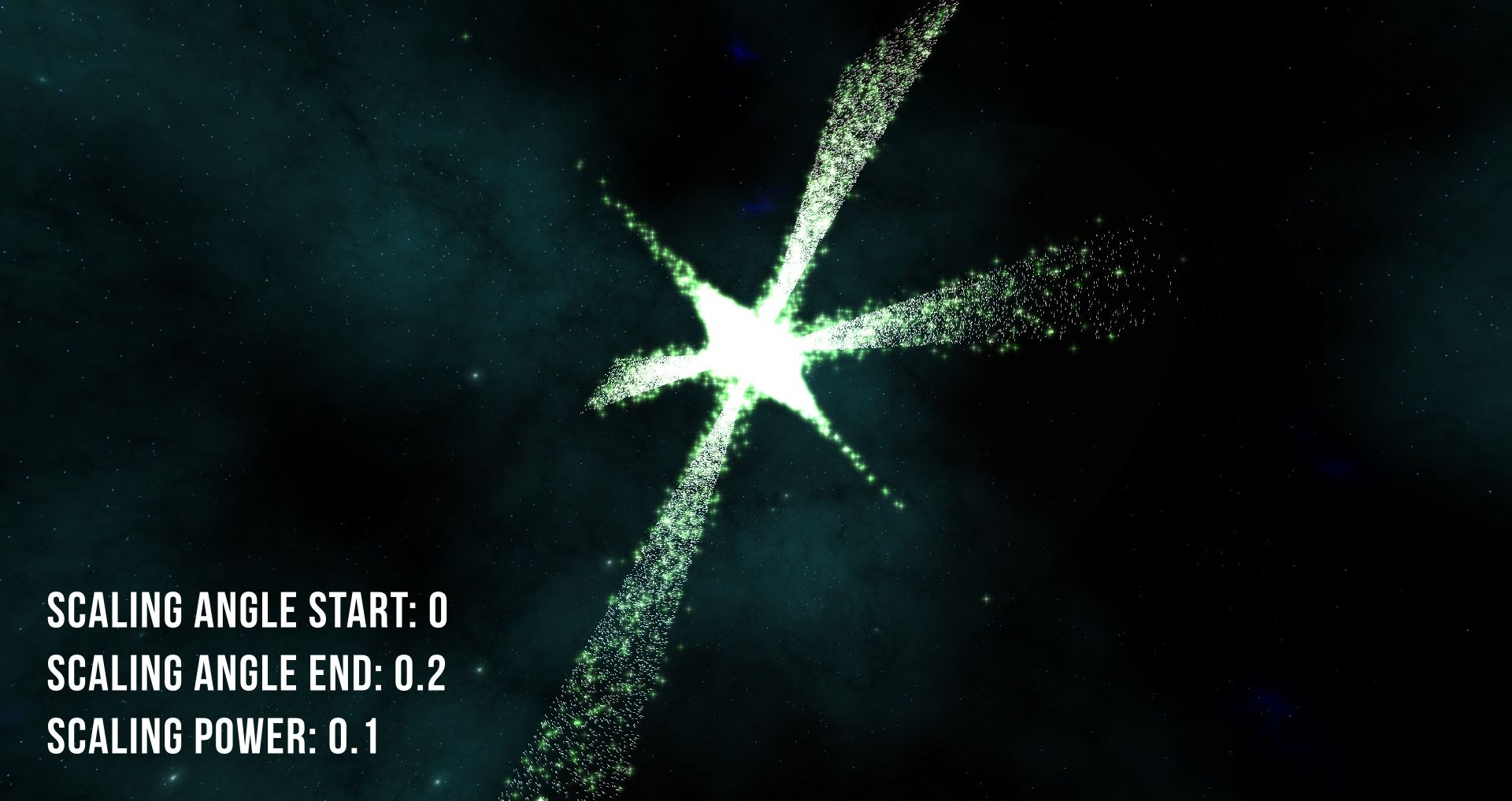The top and bottom arms have been scaled outwards

If scalingAngleEnd is set to PI, all points will land in the scaling zone: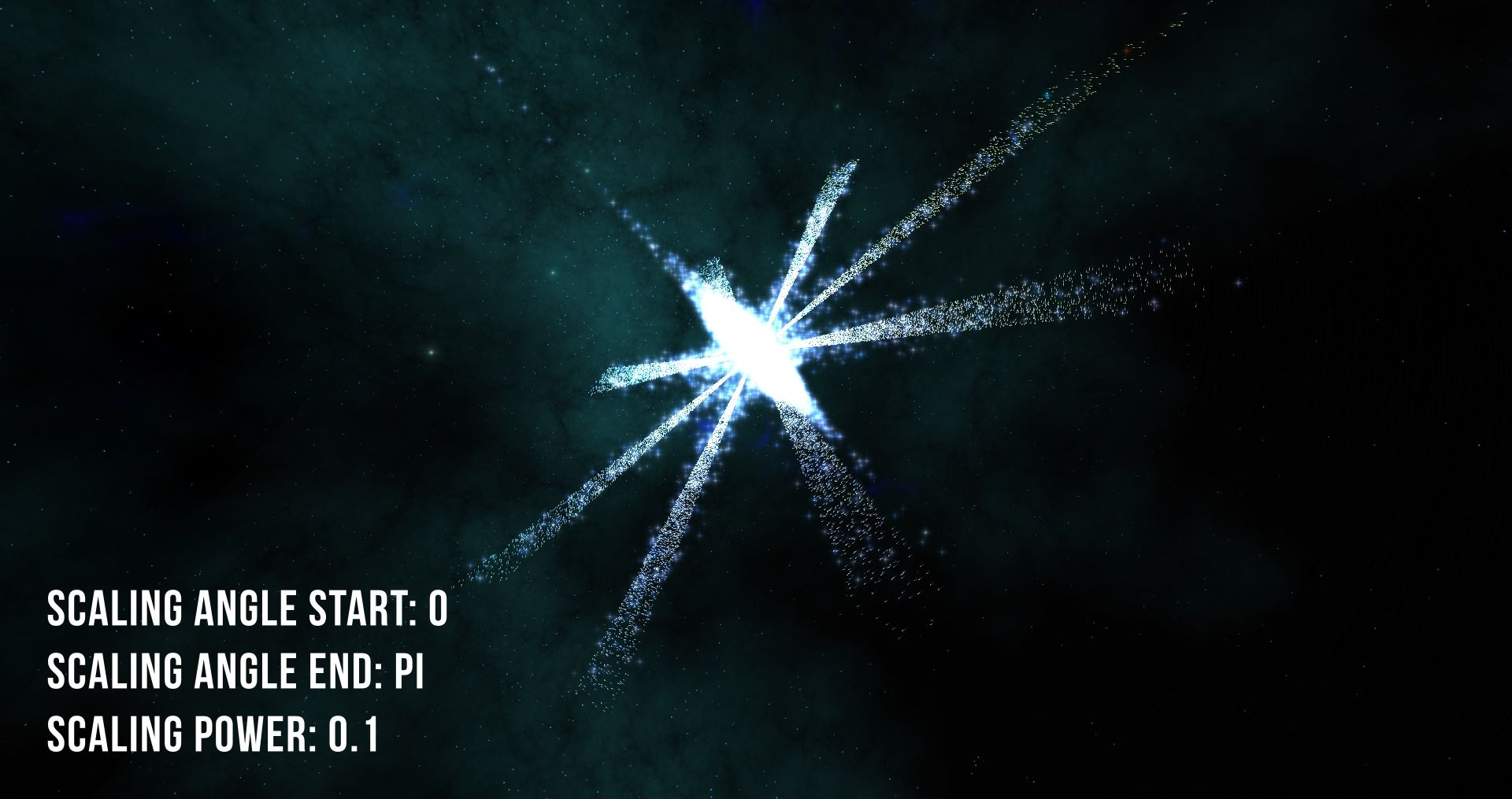All points have been scaled outwards

Part 4 - Arm Bending

To bend each arm around the galaxy we apply a matrix transformation to each point. Points further from the center of the galaxy will have a stronger rotation applied to them.

Vector3 GetPoint()
{
// Generate the point
...

// Calculate arm scaling
...

// Rotate the point around the galaxy proportional to its magnitude
v *= Matrix4.CreateRotationY(-v.Magnitude * rotationStrength);

v.Y = 0;
return v;
}

The images below show the results of changing the rotationStrength parameter. Note these examples have more arms to demonstrate the bending more clearly.

Part 5 - Vertical Variance

By default, the arms generated are flat. By setting the heightMagnitude parameter to a value greater than 0, the vertical position of each point is calculated using a sine wave:

Vector3 position = GetPoint();
position.Y -= heightMagnitude * Math.Sin(position.Magnitude * heightFrequency);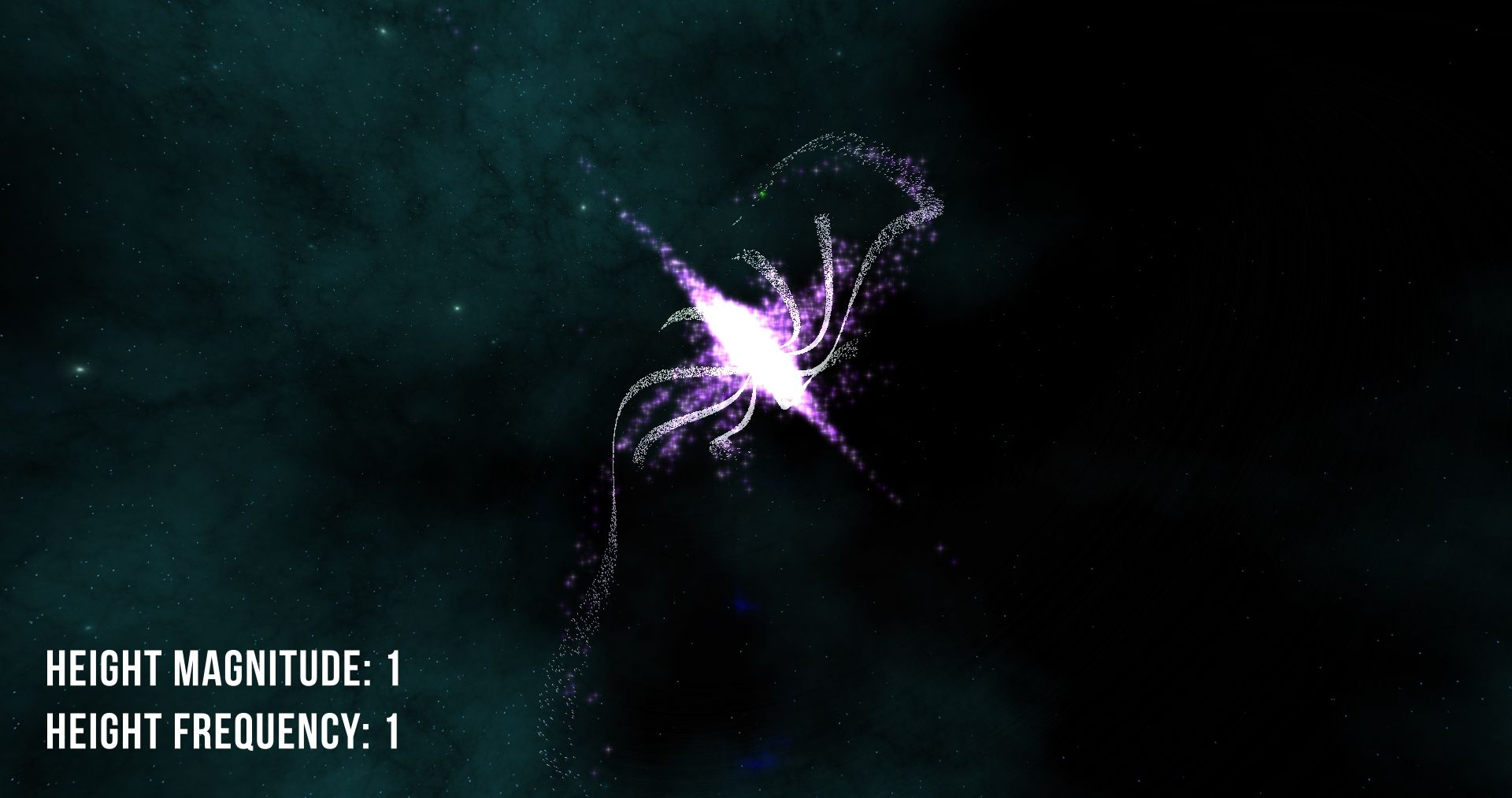The arms of this galaxy are manipulated vertically using a sin wave

Part 6 - Applying Colour

Each quadrant of the galaxy has its own colour (variables c1, c2, c3 and c4).

Once we have generated a point, its colour is calculated based on its angle and distance from the center of the galaxy.

Vector3 position = GetPoint();
position.Y -= heightMagnitude * Math.Sin(position.Magnitude * heightFrequency);

// Colour each point based on their global angle around the axis
Color blendedColour;
var angle = Math.Atan2(position.X, position.Z);

// c1, c2, c3 and c4 are random colours generated at the start of the program
// Blend the colours of adjacent quadrants together
if (angle < -Math.PI / 2)
blendedColour = Color.Interpolate(c1, c2, (angle + Math.PI) / (Math.PI / 2));
else if (angle < 0)
blendedColour = Color.Interpolate(c2, c3, (Math.PI / 2 + angle) / (Math.PI / 2));
else if (angle < Math.PI / 2)
blendedColour = Color.Interpolate(c3, c4, angle / (Math.PI / 2));
else
blendedColour = Color.Interpolate(c4, c1, (angle - Math.PI / 2) / (Math.PI / 2));

// maxMagnitude stores the distance of the furthest known point
if (position.Magnitude / maxMagnitude > 1)
maxMagnitude = position.Magnitude;

// Colours get brighter the closer they are to the center
uint pointColour = Color.Interpolate(Color.Black, Color.Interpolate(Color.White, blendedColour, position.Magnitude / maxMagnitude), 0.8).Value;

Part 7 — Multiple Arm Layers

To add depth to the galaxy, the arm creation process can be repeated multiple times. Every iteration uses a different heightMagnitude to make each arm follow a different vertical path.

// Create multiple layers
for (int o = 0; o < layers; o++)
{
// Create the arms
for (int i = 0; i < 100000; i++)
{
Vector3 position = GetPoint();
position.Y -= heightMagnitude * Math.Sin(position.Magnitude * heightFrequency);

// Colour and store each point
...
}

// Randomise the height magnitude for the next layer
// so that each layer follows a different path
heightMagnitude *= random.NextDouble();

// 50% chance that the next layer will be flipped vertically
if (random.NextDouble() > 0.5)
heightMagnitude *= -1;
}